# Octahedron

(diff) ← Older revision | Latest revision (diff) | Newer revision → (diff)

A solid figure having eight triangular faces, twelve edges and six vertices, with 4 faces at each vertex. If all edges have the same length, it is one of the five regular polyhedra (Platonic solids); if the edge length is, then the volume of the octahedron is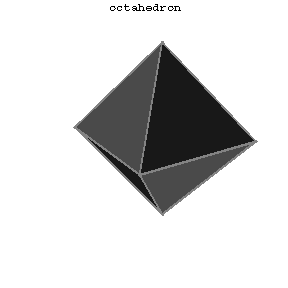Figure: o068100a

The Schläfli symbol of an octahedron is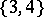. When the edges all have the same length one deals with the regular octahedron, reciprocal to the cube; it can be regarded either as a triangular anti-prism or as a square double-pyramid. As one of the five Platonic polyhedra (cf. Regular polyhedra; Platonic solids) it represents the ancient element air. It occurs in nature as a crystal of chrome alum. In terms of its circumradius as unit of measurement, its six vertices have Cartesian coordinates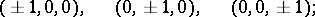thus it has edge-length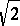, inradiusand volume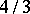. Its 4 pairs of opposite faces (or the 4 diameters of the cube) are freely permuted by the octahedral group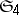of order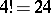.